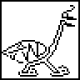The Mathematical Dialect Quiz1. What do you call a rigorous demonstration that a statement is true?
1. If “proof,” then you’re a mathematician
2. If “experiment,” then you’re a physicist
3. If you have no word for this concept, then you’re an economist1. What do you call a slow, painful, computationally intense method of solving a problem?
1. If “engineering,” then you’re a mathematician
2. If “mathematics,” then you’re an engineer
1. What do you call a person who is in their first job after a PhD?
1. If “postdoc,” then you’re a mathematician or physicist
2. If “assistant professor,” then you’re an economist
3. If “wealthy,” then you’re a computer scientist
4. If you have no word for a job after a PhD, then you’re in the humanities, and you have our condolences1. What do you call a calculator with graphing capabilities?
1. If “an antique,” then you’re a computer scientist
2. If “my precious,” then you’re an engineer
3. If “the poor man’s Wolfram Alpha,” then you’re a mathematician
4. If “kinda hard to use,” then you’re an honest mathematician1. How do you pronounce “Pythagorean”?
1. If you pronounce it “pithAGorEan,” then you’re a mathematician
2. If you pronounce it “PITHaGORean,” then you’re a physicist
3. If you just mumble the word and hope no one notices, then you’re a TA1. What name do you use for the person who invented calculus?
1. If “Leibniz,” then you’re a mathematician
2. If “Newton,” then you’re a physicist
3. If “magical wizard,” then you’re probably not ready for grad school1. What do you say after successfully proving your point beyond all doubt?
1. If “QED,” then you’re a mathematician
2. If “the prosecution rests,” then you’re a mathematician with a flair for drama
3. If you do not believe proof beyond all doubt is possible, then you’re a scientist1. What do you call a simplified representation of reality, such as imagining a physical system with no friction or air resistance?
1. If “a model,” then you’re a computer scientist
2. If “an approximation,” then you’re an engineer
3. If you call this “reality,” then you’re an economist1. How do you refer to a piece of work that suffers from one small but visible mistake?
1. If “rough,” then you’re an engineer
2. If “as good as it’s going to get,” then you’re a computer scientist
3. If “worthless,” then you’re a mathematician1. What do you call a formal gathering of professionals from your field?
1. If “a conference,” then you’re a physicist
2. If “a start-up,” then you’re a computer scientist
3. If “an advisory panel to the president,” then you’re an economist
4. If “a game of D&D,” then you’re a mathematicianThanks for reading! If you prefer bad gifs to bad drawings, you might also check out The Math Aficionado’s Guide to High Fives.

67 thoughts on “The Mathematical Dialect Quiz”

1.Aliyaaaa says:

This is brilliant! Particularly from a Mathematics undergrad’s perspective!

2.fred hartshorn says:

I was an engineer with a Math degree…

3.Amcii C. Bellamy says:

Reblogged this on Subatomic-Visage: and commented:
Like a lot…thanks for post!

4.lablua says:

“1. What do you call a rigorous demonstration that a statement is true?”

D. If “steps to reproduce” or “reproduction”, then you’re a computer scientist.

5.gand3r says:

8. What do you call a simplified representation of reality, such as imagining a physical system with no friction or air resistance?

D. If “an abstraction,” then you’re a mathematician.

6.Hélène Wilkinson says:

Wonderful, thank you so much.

5. What’s a TA?

7. Wouldn’t Gödel have answered C? He was a mathematician…